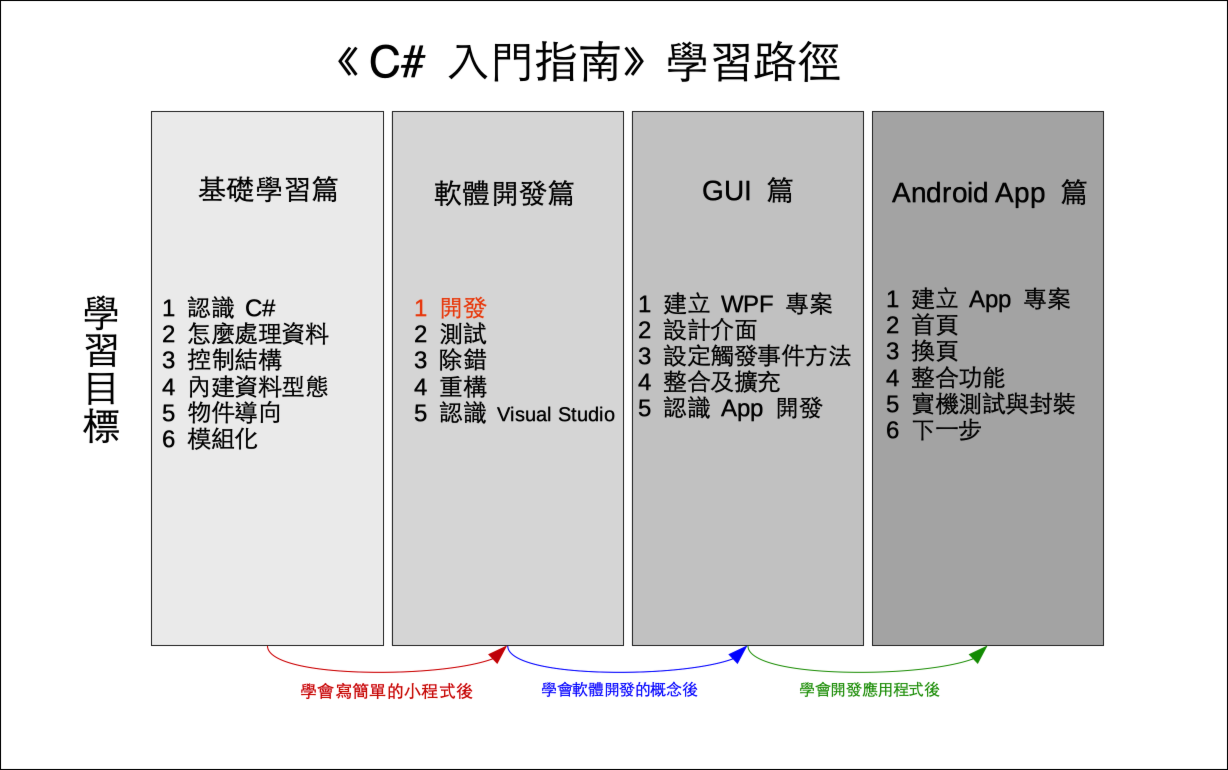# 單元 18 - 編碼

～～學習進度表～～There is no spoon.
×

 0 1 2 3 4 5 6 7 8 9 10 11 12 13 14 15 16 17 18 19 20 21 22 23 24 25 q z i r a j s b k t c l u d m v e n w f o x g p y h

``code_array = "qzirajsbktcludmvenwfoxgpyh"``

``"There is no spoon."``

``````// 編碼方法
public string ToEncode(string s)
{
// 建立暫存字串
string result = "";

char c;
int m;
// 逐一取得字元進行處理
for (int i = 0; i < s.Length; i++)
{
// 判斷是否為英文小寫字母
if (s[i] >= 97 && s[i] <= 122)
{
c = s[i];
m = c - 97;
result += code[m];
}
// 不是英文小寫字母，直接將字元附加到 result 之後
else
{
result += s[i];
}
}

// 回傳結果
return result;
}``````

ToEncode() 接收一個字串 s 當參數 (parameter) ，也回傳一個新字串 results 就是要編碼的字串，而 result 則是編碼過的字串。

``````// 逐一取得字元進行處理
for (int i = 0; i < s.Length; i++)
{
// 判斷是否為英文小寫字母
if (s[i] >= 97 && s[i] <= 122)
{
c = s[i];
m = c - 97;
result += code[m];
}
// 不是英文小寫字母，直接將字元附加到 result 之後
else
{
result += s[i];
}
}``````

``result += code[m];``

``````using System;

namespace EncryptDemo06
{
class EncryptDemo06
{
// 宣告密碼表欄位
public string code;

// 建構子，呼叫建立密碼表的 SetCode() 方法
public EncryptDemo06()
{
SetCode();
}

// 建立密碼表
public void SetCode()
{
// 宣告變數
int a, b, x, y, m;
char c = 'a';
// a 、 b 初始化
Random r = new Random();
a = 0;
b = 0;
// a 不能是 0 或偶數
while (a % 2 == 0)
{
a = r.Next(1, 10);
}
b = r.Next(0, 10);
code = "";
// 建立密碼表的迴圈
for (int i = 0; i < 26; i++)
{
x = c;
y = x * a + b;
m = y % 26;
code += Convert.ToChar(m + 97);
c++;
}
}

// 編碼方法
public string ToEncode(string s)
{
// 建立暫存字串
string result = "";

char c;
int m;
// 逐一取得字元進行處理
for (int i = 0; i < s.Length; i++)
{
// 判斷是否為英文小寫字母
if (s[i] >= 97 && s[i] <= 122)
{
c = s[i];
m = c - 97;
result += code[m];
}
// 不是英文小寫字母，直接將字元附加到 result 之後
else
{
result += s[i];
}
}

// 回傳結果
return result;
}

// 解碼方法
public string ToDecode(string s)
{
return s;
}
}

class Program
{
static void Main(string[] args)
{
// 建立密碼物件
EncryptDemo06 e = new EncryptDemo06();
// 印出密碼表
Console.WriteLine(e.code);
// 建立測試字串
string s1 = "There is no spoon.";
// 印出測試字串
Console.WriteLine(s1);
// 進行編碼
string s2 = e.ToEncode(s1);
// 印出編碼結果
Console.WriteLine(s2);
}
}
}

//《程式語言教學誌》的範例程式
// http://kaiching.org/
// 專案：EncryptDemo06
// 檔名：Program.cs
// 功能：示範利用 C# 設計 Encrypt 類別
// 作者：張凱慶``````

``````static void Main(string[] args)
{
// 建立密碼物件
EncryptDemo06 e = new EncryptDemo06();
// 印出密碼表
Console.WriteLine(e.code);
// 建立測試字串
string s1 = "There is no spoon.";
// 印出測試字串
Console.WriteLine(s1);
// 進行編碼
string s2 = e.ToEncode(s1);
// 印出編碼結果
Console.WriteLine(s2);
}``````

 C:\EncryptDemo06> dotnet run gnubipwdkryfmtahovcjqxelsz There is no spoon. Tdivi kc ta chaat. C:\EncryptDemo06>1. C# 程式設計手冊 | Microsoft Docs - 類別

1. 轉換表格利用字串來儲存，字串可用索引值存取元素，也就是字元。
2. 編碼時利用迴圈依序取得要編碼字串的所有字元，先判斷是否為英文小寫字母，如果是英文小寫字母就進行編碼，如果不是就直接把該字元附加到暫存變數的最後。
3. 加號運算子在字串型態被多載過，可用於字串連結並且重新指派。

1. 要怎麼判斷一個字元是英文小寫字母或大寫字母？
2. 多載的意義是什麼？為什麼運算子可以多載？

1. 承接上一個單元的猜數字遊戲，將新專案寫在 Exercise1801 中，在遊戲迴圈中檢查使用者輸入的長度，若是長度不等於 4 就印出提示訊息進行下一輪。
2. 承上題，將新專案寫在 Exercise1802 中，替 Guess 類別增加建構子，並且在建構子中設定三個欄位 answerab 的初值。